### לבקשת מידע ויצירת קשר בנוגע לקורסים, מלאו ושלחו את פרטיכם, ואנו נחזור אליכם בהקדם: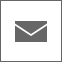## צור קשר

צוות מרכז ההדרכה ישמח לסייע לך בכל שלב בייעוץ, בהכוונה והכי חשוב ביחס אישי, חם ומקצועי.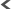03-7660111 שלוחה 6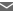טופס בקשת מידע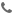יצירת קשר טלפוני## דרכי הגעה

##### רחוב ראול ולנברג 4, רמת החייל, תל אביב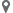מפת הגעהמפת גישה לחניה

##### אוטובוסים ורכבות

תחנת רכבת אוניברסיטה - עתידים תל אביב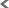קו 12קו 189קו 389

תחנת רכבת ואוטובוסים ארלוזרוב - עתידיםקו 141קו 142

תחנה מרכזית תל אביב - עתידיםקו 52

ללוחות זמנים בדקו באתר:דן תחבורה ציבוריתרכבת ישראל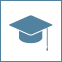## קורסים בתוכנות MATLAB & Simulink

### הקורסים מתקיימים Online או בכיתות הדרכה

#### MATLAB

MATLAB Fundamentals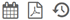מועד קרוב: 13+20+27 ביולי 2021 משך הקורס: 3 ימים סילבוס להורדה

MATLAB Fundamentals

This three-day course provides a comprehensive introduction to the MATLAB technical computing environment. The course is intended for beginning users and those looking for a review. No prior programming experience or knowledge of MATLAB is assumed. Themes of data analysis, visualization, modeling, and programming are explored throughout the course.

Topics include:

• Working with the MATLAB user interface
• Entering commands and creating variables
• Analyzing vectors and matrices
• Visualizing vector and matrix data
• Working with data files
• Working with data types
• Automating commands with scripts
• Writing programs with logic and flow control
• Writing functions

Prerequisites: Undergraduate-level mathematics and experience with basic computer operations.

MATLAB Programming Techniquesמועד קרוב: 06+13 בדצמבר 2021 משך הקורס: 2 ימים סילבוס להורדה

MATLAB Programming Techniques

This two-day course provides hands-on experience using the features in the MATLAB language to write efficient, robust, and well-organized code. These concepts form the foundation for writing full applications, developing algorithms, and extending built-in MATLAB capabilities. Details of performance optimization, as well as tools for writing, debugging, and profiling code, are covered. Topics include:

• Creating robust applications
• Utilizing development tools
• Structuring code
• Structuring data
• Efficient data management
• Classes and objects

Prerequisites: MATLAB Fundamentals

Signal Processing with MATLABטרם נקבעו מועדים. משך הקורס: 2 ימים סילבוס להורדה

Signal Processing with MATLAB

This two-day course shows how to analyze signals and design signal processing systems using MATLAB, Signal Processing Toolbox and DSP System Toolbox. Topics include:

• Creating and analyzing signals
• Performing spectral analysis
• Designing and analyzing filters
• Designing multirate filters

Prerequisites:  MATLAB Fundamentals or equivalent experience using MATLAB, and a good understanding of signal processing theory, including linear systems, spectral analysis and filter design.

Image Processing with MATLABמועד קרוב: 07+14 ביוני 2021 משך הקורס: 2 ימים סילבוס להורדה

Image Processing with MATLAB

This two-day course provides hands-on experience with performing image analysis. Examples and exercises demonstrate the use of appropriate MATLAB and Image Processing Toolbox functionality throughout the analysis process. Topics include:

• Importing and exporting images
• Analyzing images interactively
• Removing noise
• Aligning images and creating a panoramic scene
• Detecting lines and circles in an image
• Segmenting object edges
• Segmenting objects based on their color and texture
• Performing batch analysis over sets of images
• Segmenting objects based on their shape using morphological operations
• Measuring shape properties

Prerequisites: MATLAB Fundamentals or equivalent experience using MATLAB. Basic knowledge of image processing concepts is strongly recommended.

Computer Vision with MATLABתאריך קרוב: 22 ביוני 2021 משך הקורס: 1 יום סילבוס להורדה

Computer Vision with MATLAB

This one-day course provides hands-on experience with performing computer vision tasks.  Examples and exercises demonstrate the use of appropriate MATLAB® and Computer Vision Toolbox™ functionality.

Topics include:

• Importing, displaying, and annotating images and videos
• Detecting, extracting, and matching object features
• Automatically aligning images using geometric transformations
• Detecting objects in images and videos
• Tracking objects and estimating their motion in a video

Pre- requisites:

MATLAB Fundamentals or equivalent experience using MATLAB.

Image Processing with MATLAB and basic knowledge of image processing and computer vision concepts.

Deep Learningתאריך קרוב: 06 ביוני 2021 משך הקורס: 1 יום סילבוס להורדה

Deep Learning

This one-day course provides a comprehensive introduction to practical deep learning using MATLAB. Attendees will learn how to create, train, and evaluate different kinds of deep neural networks.

Topics include:

• Using convolutional neural networks (CNNs) for image classification, regression, and object detection
• Deep learning for audio signals / sequence data (LSTM networks)
• Modifying common network architectures (Transfer Learning) or creating new networks from scratch to solve custom problems
• Improving the performance of a network by modifying training options
• Deploying a trained CNN to a standalone embedded CPU/GPU or server

MATLAB to C with MATLAB Coderמועד קרוב: 01+08 בנובמבר 2021 משך הקורס: 2 ימים סילבוס להורדה

MATLAB to C with MATLAB Coder

This two-day course covers C code generation from MATLAB code using MATLAB Coder. The focus is on making existing MATLAB code compliant, generating C code that meets optimization requirements, and integrating generated code with external modules. Topics include:

• Preparing MATLAB code for code generation
• Working with fixed-size and variable-size data
• Integrating with external code
• Optimizing generated code

Prerequisites: MATLAB Fundamentals and a basic working knowledge of the C programming language.

Machine Learning with MATLABמועד קרוב: 04+11+18 באוגוסט 2021 משך הקורס: 3 ימים סילבוס להורדה

Machine Learning with MATLAB

This three-day course focuses on data analytics and machine learning techniques in

MATLAB using functionality within Statistics and Machine Learning Toolbox and

Neural Network Toolbox™. The first day of the training will be used to provide

introductory skills and know-how of preprocessing and analyzing signals for Data

Analytics applications.

Communication Systems Design with MATLABקורס מותאם אישית המועבר במסגרת פרטית בלבד וסגור לעובדי החברה המזמינה. פרטים אצל נציגי הקורסים משך הקורס: 1 יום סילבוס להורדה

Communication Systems Design with MATLAB

This one-day course shows how to design and simulate digital communication systems using MATLAB. Different channel impairments and their modeling are demonstrated.

Prerequisites: MATLAB Fundamentals and knowledge of digital communication systems.

Control System Design with MATLAB and Simulink21+28 ביוני 2021 משך הקורס: 2 ימים סילבוס להורדה

Control System Design with MATLAB and Simulink

This two-day course provides a general understanding of how to accelerate the design process for closed-loop control systems using MATLAB® and Simulink®.

Topics include:

• Control system design overview
• System modeling
• System analysis
• Control design
• Controller implementation

Accelerating and Parallelizing MATLAB Code10 ביוני 2021 משך הקורס: 1 יום סילבוס להורדה

Accelerating and Parallelizing MATLAB Code

This course covers a variety of techniques for making your MATLAB® code run faster.

You will identify and remove computational bottle-necks using techniques like preallocation and vectorization.

On top of that, you will take advantage of multiple cores on your computer by parallelizing for-loops with Parallel Computing Toolbox™.

If you are working with long-running simulations, you will benefit from the hands-on demonstrations and exercises in the course.

Topics include:

• Improving performance within core MATLAB
• Generating MEX-files
• Parallelizing computations
• GPU computing

Simulink for System and algorithm Modelingמועד קרוב: 23+30 במאי 2021 משך הקורס: 2 ימים סילבוס להורדה

Simulink for System and algorithm Modeling

This two-day course is for engineers who are new to system and algorithm modeling and design validation in Simulink. It demonstrates how to apply basic modeling techniques and tools to develop Simulink block diagrams. Topics include:

• Creating and modifying Simulink models and simulating system dynamics
• Modeling continuous-time, discrete-time, and hybrid systems
• Modifying solver settings for simulation accuracy and speed
• Building hierarchy into a Simulink model
• Creating reusable model components using subsystems, libraries, and model references

Prerequisites: MATLAB Fundamentals course or equivalent experience using MATLAB.

Signal Processing with Simulinkמועד קרוב: טרם נקבע משך הקורס: 3 ימים סילבוס להורדה

This three-day course covers basic modeling techniques and tools for developing Simulink block diagrams for signal processing applications.  Topics include:

• Modeling single-channel and multichannel discrete dynamic systems
• Implementing sample-based and frame-based processing
• Modeling mixed-signal (hybrid) systems
• Developing custom blocks and libraries
• Modeling condition-based systems
• Performing spectral analysis with Simulink
• Integrating filter designs into Simulink
• Modeling multirate systems
• Incorporating external code

Prerequisites: MATLAB Fundamentals and Signal Processing with MATLAB.

Simulink Model Management and Architectureקורס מותאם אישית המועבר במסגרת פרטית בלבד וסגור לעובדי החברה המזמינה. פרטים אצל נציגי הקורסים משך הקורס: 2 ימים סילבוס להורדה

This two-day course describes techniques for applying Model-Based Design in a common design workflow. It provides guidance on managing and sharing Simulink models when working in a large-scale project environment. Topics include:

• Implementing interface control of Simulink subsystems and models
• Managing requirements in Simulink models
• Partitioning models using Simulink subsystems, libraries, and model references
• Managing a model and all its dependencies in a project
• Controlling the location, scope, and code generation behavior of model data
• Establishing and enforcing modeling standards
• Documenting and sharing a Simulink model

Pre-requisites: MATLAB Fundamentals and Simulink for System and Algorithm Modeling. This course is intended for intermediate or advanced Simulink users.

Verification and Validation of Simulink Modelsקורס מותאם אישית המועבר במסגרת פרטית בלבד וסגור לעובדי החברה המזמינה. פרטים אצל נציגי הקורסים משך הקורס: 1 יום סילבוס להורדה

Verification and Validation of Simulink Models

This one-day course describes techniques for testing and formally verifying Simulink model behavior. Topics include:

• Recalling the role of verification and validation in Model-Based Design
• Configuring Simulink models for testing
• Testing a Simulink model for accuracy and coverage
• Formally verifying model behavior
• Publishing test results

Prerequisites: MATLAB Fundamentals and Simulink for System and Algorithm Modeling. This course is intended for intermediate or advanced Simulink users. Familiarity with creating MATLAB scripts and functions is recommended.

Communication Systems Modeling with Simulinkקורס מותאם אישית המועבר במסגרת פרטית בלבד וסגור לעובדי החברה המזמינה. פרטים אצל נציגי הקורסים משך הקורס: 1 יום סילבוס להורדה

This one-day course uses hands-on examples to demonstrate how to design end-to-end communication systems using Simulink, Communications System Toolbox™, and DSP System Toolbox. Topics include:

• Modeling a communication system
• Analyzing the bit error rate (BER) of a communication system

Prerequisites: MATLAB Fundamentals, Signal Processing with MATLAB and Signal Processing with Simulink.

Generating HDL Code from Simulinkמועד קרוב: 23+30 בנובמבר 2021 משך הקורס: 2 ימים סילבוס להורדה

This two-day course shows how to generate and verify HDL code from a Simulink model using HDL Code and HDL Verifier. Topics include:

• Preparing Simulink models for HDL code generation
• Generating HDL code and test bench for a compatible Simulink model
• Performing speed and area optimizations
• Integrating handwritten code and existing IP
• Verifying generated HDL code using test bench and cosimulation

Control System Design with MATLAB and Simulink21+28 ביוני 2021 משך הקורס: 2 ימים סילבוס להורדה

Control System Design with MATLAB and Simulink

This two-day course provides a general understanding of how to accelerate the design process for closed-loop control systems using MATLAB® and Simulink®.

Topics include:

• Control system design overview
• System modeling
• System analysis
• Control design
• Controller implementation

#### Stateflow for Logic Driven System Modeling

Stateflow for Logic Driven System Modelingקורס מותאם אישית המועבר במסגרת פרטית בלבד וסגור לעובדי החברה המזמינה. פרטים אצל נציגי הקורסים משך הקורס: 2 ימים סילבוס להורדה

Stateflow for Logic Driven System Modeling

This two-day course shows how to implement complex decision flows and finite-state machines using Stateflow. The course focuses on how to employ flow graphs, state machines, and truth tables in Simulink designs. Topics include:

• Modeling complex logic flows
• Modeling state machines
• Implementing hierarchical state machines
• Implementing multiprocessing state machines
• Using events in state charts
• Calling functions from state charts
• Implementing truth tables